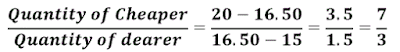IBPS Clerk 2021 Notification IBPS (Institute of Banking Personnel Selection) conducts a common recruitment process (CRP) every year to the r...

# Mixture and Alligation Shortcuts and Tricks

## Mixture and Alligation for bank PO and clerk exams

Mixture and Alligation questions shortcuts for bank exams:Almost all IBPS PO and Clerk examinations contain mixture and alligation problems.This is the area from where you can easily score few marks by understanding some formulas and shortcuts.Here we are sharing some important mixture and alligation problem formulas and tricks.If two different commodities, one of which is cheaper than the other, are mixed to obtain a new mixture, Cost Price of unit value of this new mixture is called mean price.

### Basic formula for mixture and alligation problem

If two different commodities, one of which is cheaper than the other, are mixed to obtain a new mixture, Cost Price of unit value of this new mixture is called mean price.This is the the first and foremost formula you should remember to solve mixture and alligation questions.

Example:A merchant blends two types of rice costing Rs.15 per kg and Rs.20 per kg .In what ratio should these two rice to be mixed so that resulting mixture may cost Rs.16.50 per kg.Note:
When water is mixed in milk or any liquid in such away that resulting mixture gives a profit of x% when sold at C.P of milk/liquid. Then ratio of Quantity water:Quantity of milk=x:100
Eg: In what ratio should water be mixed in milk so that seller makes a profit of 10% when mixture is sold at cost price of milk? Water : milk =10:100=1:10.

### Solving mixture and alligation problems (If more than two different commodities are mixed)

Example:If A cost 95 per kg,B cost 60 per kg,C cost 90 per kg and D cost 50 per kg. They are blended in such a way that the cost price of resulting mixture is 80.In what ratio four commodities are mixed?
To solve these kind of problems follow the steps below
Step1: Arrange them in ascending order
50
60
90
95
Step2: Make couples ,one is above mean price and other is below mean price

Step3:Now find difference between price and mean price and write it opposite to the price linked to it.

Step3: Required ratio Qt of A: Qt of B: Qt of C: Qt of D=15:10:20:30=3:2:4:6

Note:

In ‘n’ equal sized vessels two liquid P and Q are filled in the ratio p1:q1,p2:q2,p3:q3……….. pn:qn respectively
When they are mixed,

If vessels are of different quantities say x1,x2,x3….xn.

### Removal and replacement problems

A vessel contains x litre of milk. y litre is drawn and replaced by water.Then again y litre of solution is replaced by water. If this process is repeated ‘n’ times,then

Example:9 litre are drawn from a cask full of milk and then filled with water.9 litre of mixture are drawn and cask is again filled with water.Quantity of milk now left in the cask is to that of water in at is as 16:9.What is the capacity of cask in litre?
Ans:let x be the capacity of cask which is=quantity of initial milk,### Mixture and alligation practice questions for bank tests

Qs1.In what ratio must a seller mix two varieties of pulses costing Rs. 12 and Rs. 20 per kg respectively so as to get a mixture worth Rs.15 ?
A. 5 : 7
B. 5 : 3
C. 7 : 3
D. 3 : 5

Qs2.How many kilogram of sugar costing Rs. 9 per kg must be mixed with 27 kg of sugar costing Rs. 7 per kg so that there may be a gain of 10% by selling the mixture at Rs. 9.24 per kg?
A. 63kg
B. 72 kg
C. 57 kg
D. 56kg

Qs3. A container contains 40 litres of milk. From this container 4 litres of milk was taken out and replaced by water. This process was repeated further two times. How much milk is now contained by the container?
A.26.90 litres
B.29.16 litres
C.27.55 litres
D.30.70litres

Qs4.A and B are two alloys of gold and copper prepared by mixing these metals in the ratio 7:2 and 7:11 respectively. If equal quantities of alloys are melted to form a third alloy C the ratio of gold and copper in the alloy C?
A.5:7
B.7:5
C.2:5
D.5:2

Qs.5.13. How much water must be added to 10 ltr of 80% solution acid to reduce it to a 50% solution ?
A.20 ltr
B.40 ltr
C.80 ltr
D.60 ltr

If you find this Mixture and alligation formulas and tricks for bank exams useful share it
[mixture and alligation tricks pdf,mixture and alligation problems tricks,mixture and alligation short tricks mixture and alligation formula,mixture and alligation solved problems,mixture and,alligation concepts,alligation mixture tutorial,mixture and alligation]